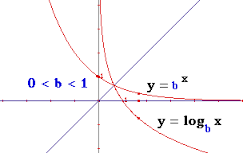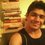# Something crazy & New to learn !All the wonder Brilliant users and all my followers, this day I am going to share something really very interesting and may be something new for many of you.

Inspired from a question by @Calvin Lin sir, I have got some discussion points in my mind.

Hope, you all are aware of functions (make mathematics awesome and easy). Here I would like to talk about the function itself and its inverse.

Inverse of a function will exist, if and only if it is one-one(injective) function and onto (surjective) function. Combining one-one and onto together, the function is called Bijective function.

There comes a property of inverse functions :

Graphs of $y=f(x)$ and $y=f^{-1}(x)$ are symmetrical about the line $y=x$ and intersect on the line $y=x$.

OR

$f(x)=f^{-1}(x)=x$ whenever graphs intersect.

Now here are some points (questions) :

1. So if we talk about $f(x)=(\frac{1}{16})^x$ and its inverse functions $y=log_{\frac{1}{16}}x$. How many points of intersection will be there ? The above property says that there will be only 1 solution of $log_{\frac{1}{16}}x=(\frac{1}{16})^x$ But algebraically two solutions $x=\frac{1}{4} and x=\frac{1}{2}$ So now the point is exactly how many roots are there for the equation $log_{\frac{1}{16}}x=(\frac{1}{16})^x$

2. Is the above stated property wrong ?

3. Can there be functions for which $f(x)=f^{-1}(x)$ has solutions except which lie on the line $y=x$. If yes, then finite or infinite number ?

4. Why does the equation $log_{\frac{1}{16}}x=(\frac{1}{16})^x$ not follow the above property ?

Note: You can add more discussion points here and analyse it as much as you can.

Thank you !

## ------------------------------------------------

###### Check out more problems which can solved easily by sketching their graphs, instead of going algebraically. So, try the set : Can you draw its graph ?Note by Sandeep Bhardwaj
6 years, 9 months ago

This discussion board is a place to discuss our Daily Challenges and the math and science related to those challenges. Explanations are more than just a solution — they should explain the steps and thinking strategies that you used to obtain the solution. Comments should further the discussion of math and science.

When posting on Brilliant:

• Use the emojis to react to an explanation, whether you're congratulating a job well done , or just really confused .
• Ask specific questions about the challenge or the steps in somebody's explanation. Well-posed questions can add a lot to the discussion, but posting "I don't understand!" doesn't help anyone.
• Try to contribute something new to the discussion, whether it is an extension, generalization or other idea related to the challenge.

MarkdownAppears as
*italics* or _italics_ italics
**bold** or __bold__ bold
- bulleted- list
• bulleted
• list
1. numbered2. list
1. numbered
2. list
Note: you must add a full line of space before and after lists for them to show up correctly
paragraph 1paragraph 2

paragraph 1

paragraph 2

[example link](https://brilliant.org)example link
> This is a quote
This is a quote
    # I indented these lines
# 4 spaces, and now they show
# up as a code block.

print "hello world"
# I indented these lines
# 4 spaces, and now they show
# up as a code block.

print "hello world"
MathAppears as
Remember to wrap math in $$ ... $$ or $ ... $ to ensure proper formatting.
2 \times 3 $2 \times 3$
2^{34} $2^{34}$
a_{i-1} $a_{i-1}$
\frac{2}{3} $\frac{2}{3}$
\sqrt{2} $\sqrt{2}$
\sum_{i=1}^3 $\sum_{i=1}^3$
\sin \theta $\sin \theta$
\boxed{123} $\boxed{123}$

Sort by:

Am I missing something? Where in property saying that there will be only 1 solution?

Sure it states that $f(x)$ and $f^{-1}(x)$ intersect on the line $y=x$, but that it. It doesn't say $f(x)$ and $f^{-1}(x)$ ONLY intersect on line $y=x$.

$f(x),~f^{-1}(x)$ and $y=x$ intersect at $x\approx0.3642$

So $f(x)=\left(\dfrac{1}{16}\right)^x$ follows this property.

Though this property is not always true (see Sandeep Bhardwaj's comment for counterexample), it is true for all real bijective functions.

- 6 years, 9 months ago

What I think is that the property stated above is not true, but this property needs to be modified. And it should be that if $f(x)=x \space \implies f^{-1}(x)=x \space \implies f(x)=f^{-1}(x)=x$. It is not saying that every $f(x)$ and $f^{-1}(x)$ intersect on the line $y=x$ only. The graphs of $f(x)$ and $f^{-1}(x)$ may intersect at points not lying on $y=x$ and not necessary that these will cut at $y=x$.

- 6 years, 9 months ago

Let's see if we can find some more example of functions having this sort of rebellion nature. @Sandeep Bhardwaj

- 6 years, 9 months ago

I have a lot of examples contradicting the above property. And one of being is take any line perpendicular to the line $y=x$ .For instance, inverse of $y=-x$ is the same $y=-x$. So here $f(x)=f^{-1}(x)$ has infinitely many solutions $i.e. every Real Number$. I think the property stated in the note is false.

Also there are many examples against the property, even though if we don't take it perpendicular to the line $y=x$.

I would like to share one example here :

$f(x)=$$\begin{cases} x+4 ,if \space x\in[1,2] \\-x+7, if \space x\in[5,6]\ \end{cases}$

$f^{-1}(x)=$$\begin{cases} x-4 ,if \space x\in[5,6]\\7-x, if \space x\in[1,2]\ \end{cases}$

Here graphs of $y=f(x)$ and $y=f^{-1}(x)$ intersect at $(\frac{3}{2},\frac{11}{2})$ and $(\frac{11}{2},\frac{3}{2})$ and Also the intersection points don't lie on the line $y=x$

- 6 years, 9 months ago

Any function that satisfies $f^2(x) = x$, will be a good example. This is because we have $x = f(y), y = f(x)$ always, and so there are many many solutions.

Apart from $f(x) = x$, the next easiest ones to find are $f(x) = - x$, and then $f(x) = - x + c$.
You might find $f(x) = \frac{1}{x}$ and $f(x) = \frac{ c x - c^2 + 1 } { x - c }$.

Staff - 6 years, 9 months ago

I think the only property we can talk about is that if we have an ecuation we can write as $f(x)=g(x)$ the solutions are the values of $x$ where the graph of $f(x)$ and $g(x)$ have intersections. When we have the coincidence that $g(x)=f^{-1}(x)$ we can be sure that some of the solutions will be the intersection points of $y=f(x)$ with $y=x$. Like in this problem

- 5 years, 11 months ago

Checkout the th function- (-x^3 +1) it have 4 points of intersection other than that with y=x

- 4 years, 11 months ago